# RS Aggarwal Solutions Class 10 Chapter 14 Height and Distance MCQ

## RS Aggarwal Solutions Class 10 Chapter 14 Height and Distance MCQ

These Solutions are part of RS Aggarwal Solutions Class 10. Here we have given RS Aggarwal Solutions Class 10 Chapter 14 Height and Distance MCQ.

Other Exercises

Question 1.
Solution: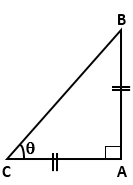Correct option: (c)
Let AB be the pole and let AC be its shadow.
Now, AB = AC
If θ is the angle of elevation of the sun, then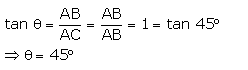Question 2.
Solution: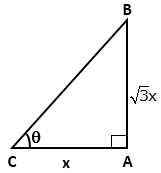Correct option: (c)
Let AB be the pole and let AC be its shadow.
Let the length of the shadow = x m.
Then, height of the pole = √3x m.
If e is the angle of elevation of the sun, then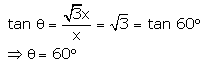Question 3.
Solution: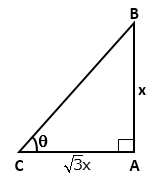Question 4.
Solution: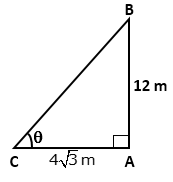Question 5.
Solution:
Correct option: (d)
Let the length of a shadow of 12-rn-high tree = x m
Now,
Ratio of lengths of objects = Ratio of lengths of their shadow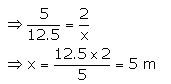Question 6.
Solution: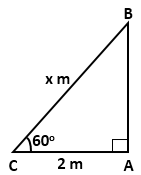Question 7.
Solution: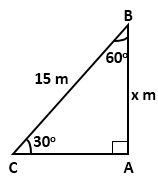Question 8.
Solution: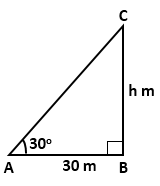Question 9.
Solution: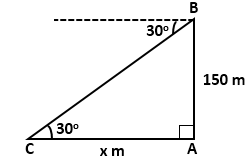Question 10.
Solution: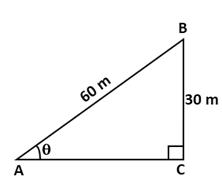Question 11.
Solution: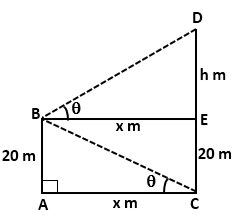Question 12.
Solution: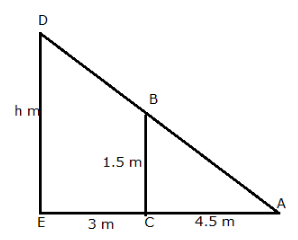Question 13.
Solution: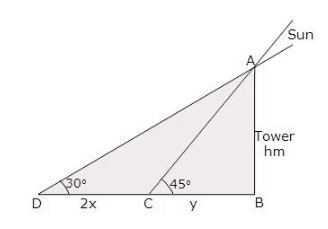Question 14.
Solution: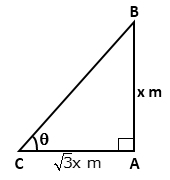Question 15.
Solution: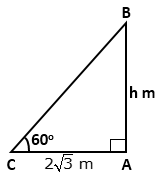Question 16.
Solution:Question 17.
Solution: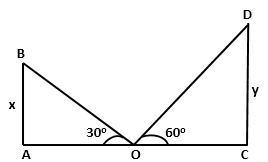Question 18.
Solution:Question 19.
Solution: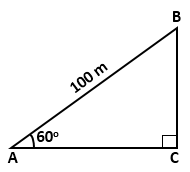Question 20.
Solution: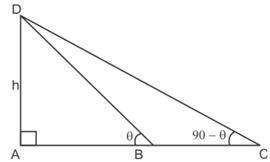Question 21.
Solution: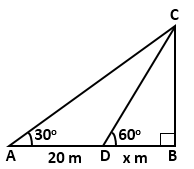Question 22.
Solution: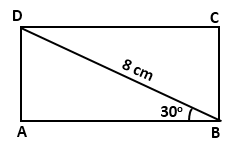Question 23.
Solution: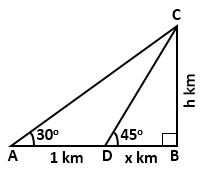Question 24.
Solution: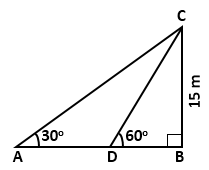Question 25.
Solution: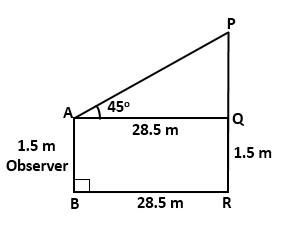Hope given RS Aggarwal Solutions Class 10 Chapter 14 Height and Distance MCQ are helpful to complete your math homework.

If you have any doubts, please comment below. Learn Insta try to provide online math tutoring for you.